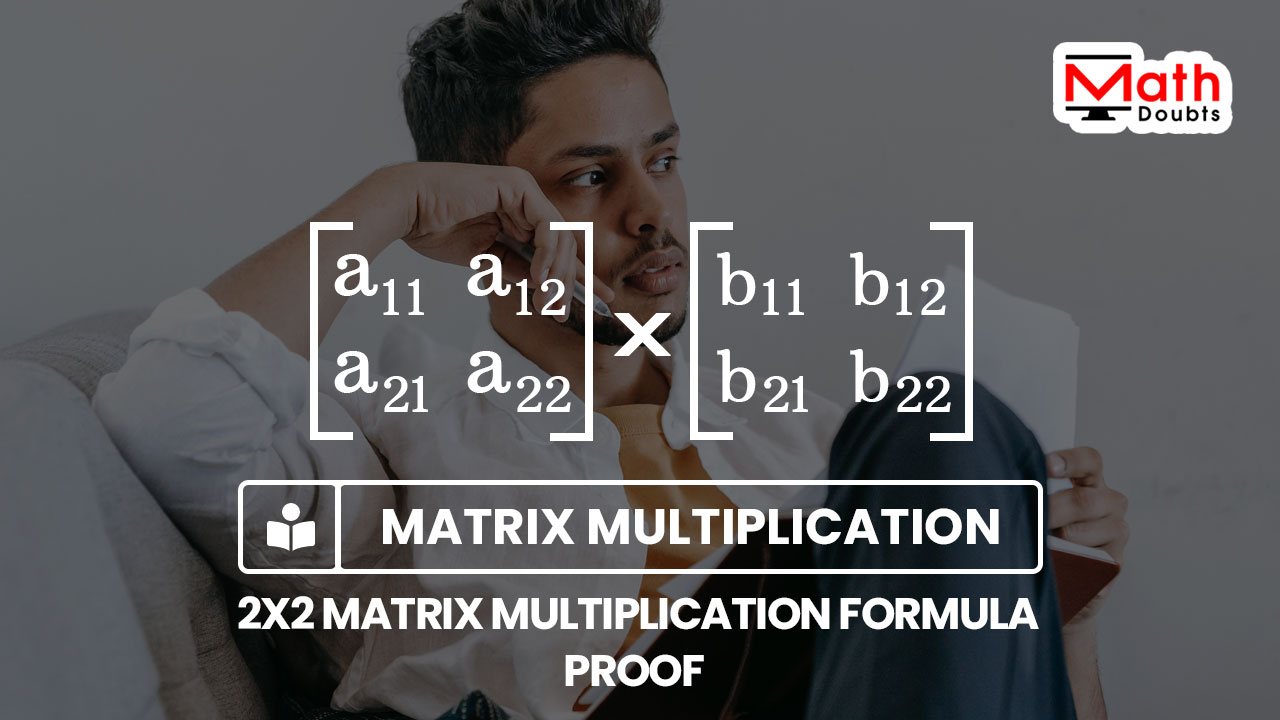# Proof of Multiplying the 2×2 Matrices formula

The multiplication of two $2 \times 2$ matrix is expressed mathematically in algebraic form as follows.

${\begin{bmatrix} a_{11} & a_{12} \\ a_{21} & a_{22} \\ \end{bmatrix}}$ $\times$ ${\begin{bmatrix} b_{11} & b_{12} \\ b_{21} & b_{22} \\ \end{bmatrix}}$Now, let’s learn how to derive a formula for the multiplication of two by two matrix by using the procedure of finding the two by two matrix multiplication in matrix algebra.

### Find sum after Multiplying the first row by first column

$\left[\begin{array}{rr} \hline a_{11} & a_{12} \\ \hline a_{21} & a_{22} \\ \end{array}\right]$ $\times$ $\left[\begin{array}{|r|r} b_{11} & b_{12} \\ b_{21} & b_{22} \end{array}\right]$

Consider the entries in first row of the first matrix and they are $a_{11}$ and $a_{12}$. Similarly, consider the entries in the first column of the second matrix and they are $b_{11}$ and $b_{21}$.

1. Multiply the elements $a_{11}$ and $a_{12}$ by the entries $b_{11}$ and $b_{21}$ respectively.
2. Add the product of $a_{11}$ and $b_{11}$ to the product of $a_{12}$ and $b_{21}$.

The sum of the products is obtained due to the multiplication of the entries in the first row of first matrix by the entries in the first column of the second matrix. So, the sum of them should be written as an entry in the first row and first column of the matrix.

$\implies$ $\left[\begin{array}{rr} \hline a_{11} & a_{12} \\ \hline a_{21} & a_{22} \\ \end{array}\right]$ $\times$ $\left[\begin{array}{|r|r} b_{11} & b_{12} \\ b_{21} & b_{22} \end{array}\right]$ $\,=\,$ ${\begin{bmatrix} a_{11} \times b_{11}+a_{12} \times b_{21} & \,\,\, \\ \,\,\, & \,\,\, \\ \end{bmatrix}}$

$\,\,=\,$ ${\begin{bmatrix} a_{11}b_{11}+a_{12}b_{21} & \,\,\, \\ \,\,\, & \,\,\, \\ \end{bmatrix}}$

### Find sum after Multiplying the first row by second column

$\left[\begin{array}{rr} \hline a_{11} & a_{12} \\ \hline a_{21} & a_{22} \\ \end{array}\right]$ $\times$ $\left[\begin{array}{r|r|} b_{11} & b_{12} \\ b_{21} & b_{22} \end{array}\right]$

In this step, consider the elements in first row of the first matrix and they are $a_{11}$ and $a_{12}$. Similarly, consider the entries in the second column of the second matrix and they are $b_{12}$ and $b_{22}$.

1. Multiply the entries $a_{11}$ and $a_{12}$ by the elements $b_{12}$ and $b_{22}$ respectively.
2. Add the product of $a_{11}$ and $b_{12}$ to the product of $a_{12}$ and $b_{22}$.

The sum of the products is evaluated due to the multiplication of the elements in the first row of first matrix by the elements in the second column of the second matrix. Therefore, the sum of them should be written as an entry in the first row and second column of the matrix.

$\implies$ $\left[\begin{array}{rr} \hline a_{11} & a_{12} \\ \hline a_{21} & a_{22} \\ \end{array}\right]$ $\times$ $\left[\begin{array}{r|r|} b_{11} & b_{12} \\ b_{21} & b_{22} \end{array}\right]$ $\,=\,$ ${\begin{bmatrix} a_{11}b_{11}+a_{12}b_{21} & a_{11} \times b_{12}+a_{12} \times b_{22} \\ \,\,\, & \,\,\, \\ \end{bmatrix}}$

$\,\,=\,$ ${\begin{bmatrix} a_{11}b_{11}+a_{12}b_{21} & a_{11}b_{12}+a_{12}b_{22} \\ \,\,\, & \,\,\, \\ \end{bmatrix}}$

### Find sum after Multiplying the second row by first column

$\left[\begin{array}{rr} a_{11} & a_{12} \\ \hline \hline a_{21} & a_{22} \\ \hline \end{array}\right]$ $\times$ $\left[\begin{array}{|r|r} b_{11} & b_{12} \\ b_{21} & b_{22} \end{array}\right]$

Now, consider the entries in second row of the first matrix and they are $a_{21}$ and $a_{22}$. Similarly, consider the elements in the first column of the second matrix and they are $b_{11}$ and $b_{21}$.

1. Multiply the elements $a_{21}$ and $a_{22}$ by the elements $b_{11}$ and $b_{21}$ respectively.
2. Add the product of $a_{21}$ and $b_{11}$ to the product of $a_{22}$ and $b_{21}$.

The sum of the products is actually calculated due to the multiplication of the entries in the second row of first matrix by the elements in the first column of the second matrix. Hence, the sum of them should be written as an entry in the second row and first column of the matrix.

$\implies$ $\left[\begin{array}{rr} a_{11} & a_{12} \\ \hline a_{21} & a_{22} \\ \hline \end{array}\right]$ $\times$ $\left[\begin{array}{|r|r} b_{11} & b_{12} \\ b_{21} & b_{22} \end{array}\right]$ $\,=\,$ ${\begin{bmatrix} a_{11}b_{11}+a_{12}b_{21} & a_{11}b_{12}+a_{12}b_{22} \\ a_{21} \times b_{11}+a_{22} \times b_{21} & \,\,\, \\ \end{bmatrix}}$

$\,\,=\,$ ${\begin{bmatrix} a_{11}b_{11}+a_{12}b_{21} & a_{11}b_{12}+a_{12}b_{22} \\ a_{21}b_{11}+a_{22}b_{21} & \,\,\, \\ \end{bmatrix}}$

### Find sum after Multiplying the second row by second column

$\left[\begin{array}{rr} a_{11} & a_{12} \\ \hline \hline a_{21} & a_{22} \\ \hline \end{array}\right]$ $\times$ $\left[\begin{array}{r|r|} b_{11} & b_{12} \\ b_{21} & b_{22} \end{array}\right]$

Finally, consider the elements in second row of the first matrix and they are $a_{21}$ and $a_{22}$. Similarly, consider the elements in the second column of the second matrix and they are $b_{12}$ and $b_{22}$.

1. Multiply the entries $a_{21}$ and $a_{22}$ by the entries $b_{12}$ and $b_{22}$ respectively.
2. Add the product of $a_{21}$ and $b_{12}$ to the product of $a_{22}$ and $b_{22}$.

The sum of the products is evaluated due to the multiplication of the elements in the second row of first matrix by the entries in the second column of the second matrix. So, the sum of them should be written as an element in the second row and second column of the matrix.

$\implies$ $\left[\begin{array}{rr} a_{11} & a_{12} \\ \hline a_{21} & a_{22} \\ \hline \end{array}\right]$ $\times$ $\left[\begin{array}{r|r|} b_{11} & b_{12} \\ b_{21} & b_{22} \end{array}\right]$ $\,=\,$ ${\begin{bmatrix} a_{11}b_{11}+a_{12}b_{21} & a_{11}b_{12}+a_{12}b_{22} \\ a_{21}b_{11}+a_{22}b_{21} & a_{21} \times b_{12}+a_{22} \times b_{22} \\ \end{bmatrix}}$

$\,\,=\,$ ${\begin{bmatrix} a_{11}b_{11}+a_{12}b_{21} & a_{11}b_{12}+a_{12}b_{22} \\ a_{21}b_{11}+a_{22}b_{21} & a_{21}b_{12}+a_{22}b_{22} \\ \end{bmatrix}}$

#### Conclusion

The multiplication formula of two by two square matrix is written in algebraic form as follows.

$\therefore\,\,\,$ ${\begin{bmatrix} a_{11} & a_{12} \\ a_{21} & a_{22} \\ \end{bmatrix}}$ $\times$ ${\begin{bmatrix} b_{11} & b_{12} \\ b_{21} & b_{22} \\ \end{bmatrix}}$ $\,=\,$ ${\begin{bmatrix} a_{11}b_{11}+a_{12}b_{21} & a_{11}b_{12}+a_{12}b_{22} \\ a_{21}b_{11}+a_{22}b_{21} & a_{21}b_{12}+a_{22}b_{22} \\ \end{bmatrix}}$

Latest Math Topics
Jun 26, 2023
Jun 23, 2023

Latest Math Problems
Jul 01, 2023
Jun 25, 2023
###### Math Questions

The math problems with solutions to learn how to solve a problem.

Learn solutions

Practice now

###### Math Videos

The math videos tutorials with visual graphics to learn every concept.

Watch now

###### Subscribe us

Get the latest math updates from the Math Doubts by subscribing us.